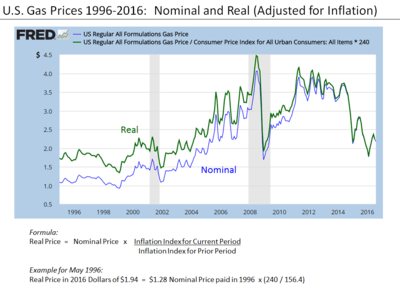# Real versus nominal value (economics)

For other uses, see Real versus nominal value.

In economics, real value is relative to other commodities or goods. It is adjusted for inflation, enabling comparison of quantities as if prices had not changed. Changes in real terms therefore exclude the effect of inflation. In contrast with real value, nominal value is not adjusted for inflation, and so increases in nominal value reflect the effect of inflation.

## Prices and inflation

A representative collection of goods, or commodity bundle, is used for comparison purposes, to measure inflation. The nominal (unadjusted) value of the commodity bundle in a given year depends on prices current at the time, whereas the real value of the commodity bundle, if it is truly representative, remains the same. The real value of individual goods or commodities may rise or fall against each other, in relative terms, but a representative commodity bundle as a whole retains its real value as a constant over time.

A price index is calculated relative to a base year. Indices are typically normalized at 100 in the base year. Starting from a base (or reference) year, a price index Pt represents the price of the commodity bundle over time t. In base year zero, P0 is set to 100. If for example the base year is 1992, real values are expressed in constant 1992 dollars, based at 100 for 1992. Also imagine for example, that the price of the commodity bundle has increased in the first year by 1%, then Pt rises from P0 = 100 to P1 = 101.

The inflation ratebetween year t - 1 and year t is:

change in price / price in year t - 1## Real valueComparison of real and nominal gas prices 1996 to 2016, illustrating the formula for conversion.

The price index is applied to adjust the nominal value of a quantity, such as wages or total production, to obtain its real value. The real value is the value expressed in terms of purchasing power in the base year.

The index price divided by its base-year valuegives the growth factor of the price index.

Real values can be found by dividing the nominal value by the growth factor of a price index. Using the price index growth factor as a divisor for converting a nominal value into a real value, the real value in year t relative to the index Pt is:Alternatively, to express a prior nominal amount in current dollars, the nominal amount is multiplied by the current value of the index price divided by the value of the index price in the prior period.

## Real growth rate

The real growth rateis the change from one period to the next of a nominal quantityin real terms. It measures by how much the buying power of the quantity has changed.where:is the nominal growth rate of,

and:is the inflation rate.For values ofbetween -1 and 1, we have the Taylor seriessoHence as a first-order (i.e. linear) approximation,## Real wages and real gross domestic product

The bundle of goods used to measure the Consumer Price Index (CPI) is applicable to consumers. So for wage earners as consumers, an appropriate way to measure real wages is to divide the nominal wage (after-tax) by the growth factor in the CPI.

Gross domestic product (GDP) is a measure of aggregate output. Nominal GDP in a particular period reflects prices which were current at the time, whereas real GDP compensates for inflation. Price indices and the U.S. National Income and Product Accounts are constructed from bundles of commodities and their respective prices. In the case of GDP, a suitable price index is the GDP price index. In the U.S. National Income and Product Accounts, nominal GDP is called GDP in current dollars (that is, in prices current for each designated year), and real GDP is called GDP in [base-year] dollars (that is, in dollars that can purchase the same quantity of commodities as in the base year).

## Example

 If for years 1 and 2 (possibly a span of 20 years apart), the nominal wage and price level P of goods are respectively nominal wage rate: $10 in year 1 and$16 in year 2 price level: 1.00 in year 1 and 1.333 in year 2, then real wages using year 1 as the base year are respectively: $10 (=$10/1.00) in year 1 and $12 (=$16/1.333) in year 2. The real wage each year measures the buying power of the hourly wage in common terms. In this example, the real wage rate increased by 20 percent, meaning that an hour's wage would buy 20% more goods in year 2 compared with year 1.

## Real interest rates

Main article: real interest rate

As was shown in the section above on real growth rate,whereis the rate of increase of a quantity in real terms,is the rate of increase of the same quantity in nominal terms, andis the rate of inflation,

and as a first-order approximation,In the case whereis a (nominal) rate of interest, andis the corresponding real interest rate, this first-order approximationis known as the Fisher equation.

Looking back into the past, the ex post real interest rate is approximately the historical nominal interest rate minus inflation. Looking forward into the future, the expected real interest rate is approximately the nominal interest rate minus the expected inflation rate.

## Cross-sectional comparison

Not only time-series data, as above, but also cross-section data which depends on prices which may vary geographically for example, can be adjusted in a similar way. For example, the total value of a good produced in a region of a country depends on both the amount and the price. To compare the output of different regions, the nominal output in a region can be adjusted by repricing the goods at common or average prices.